#### Combine

##### Description

Combine[expr]puts terms in a sum over a common denominator,and cancels factors in the result. Combine is similar to Together, but accepts the option Expanding and works usually better than Together for polynomials involving rationals with sums in the denominator.

``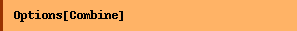``
`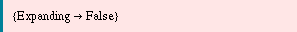`

##### Examples
``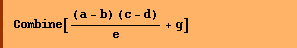``
`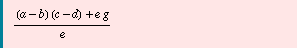`

Here the result from Together where the numerator is automatically expanded.

``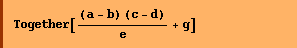``
`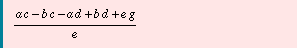`

If the option Expanding is set to True, the result of Combine is the same as Together, but uses a slightly different algorithm.

````
``

Converted from the Mathematica notebook Combine.nb